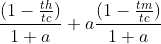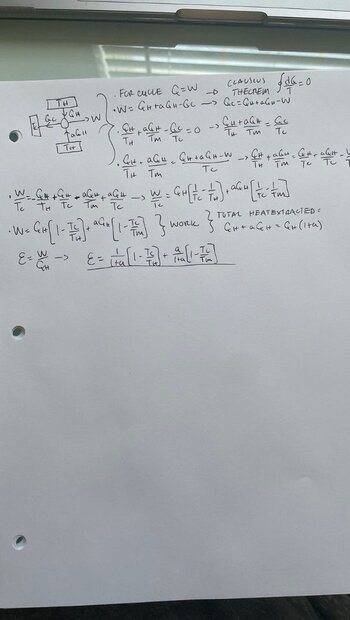# Finding an expression for the efficiency of a heat engine

• guyvsdcsniper
In summary, the conversation discusses a problem related to finding the efficiency of a heat engine. The speaker is struggling to identify where their mistake is and asks for help. They mention that the answer key provided does not make sense and that a key factor in finding the correct answer is letting the cold reservoir have a temperature of 0 K. Another person confirms the original speaker's result and points out that the book's answer always results in a negative efficiency, which is not possible.

#### guyvsdcsniper

Homework Statement
A reversible heat engine extracts
heat QH > 0 from a reservoir at temperature TH and heat QM = aQH > 0
from a reservoir at temperature TM ≤ TH while rejecting waste heat QC > 0
to a reservoir at temperature TC ≤ TM.

Derive an expression for the effi ciency of this three- reservoir heat
engine in terms of a and the three temperatures TH, TM, and TC ,
where the effi ciency is the total work produced divided by the total
heat extracted from the two hotter reservoirs.
Relevant Equations
(1 − TH /TC )/(1 + a) + (1 − TM /TC )a/(1 + a)
My book states the answer to this problem is.

I have gotten very close to the answer. My problem is my Tc/th and tc/tm are flipped compared to the solution.

I feel like I am missing something in my algebra but can't see where I am going wrong. Could I get some help identifying where my mistake is.The answer key wherever it's coming from doesn't make sense. Remember if the cold reservoir has 0 K, you are supposed to get 100% efficiency which is not happening with the answer key you cited.

•vela, Steve4Physics and guyvsdcsniper
Thank you. I was breaking my head over this but letting Tc = 0k really does help illuminate the answer

Another handy check is to let ##a=0##. This gives a simple heat engine working between ##T_h## and ##T_c##. The efficiency should then be ##1 - \frac {T_c}{T_h}## (standard formula).

•hutchphd